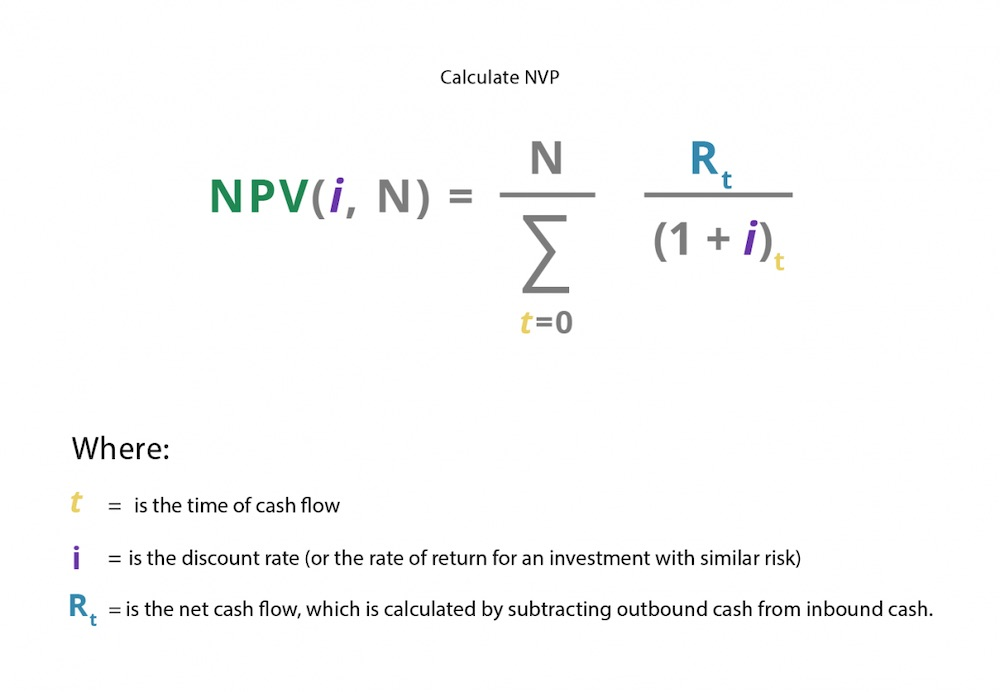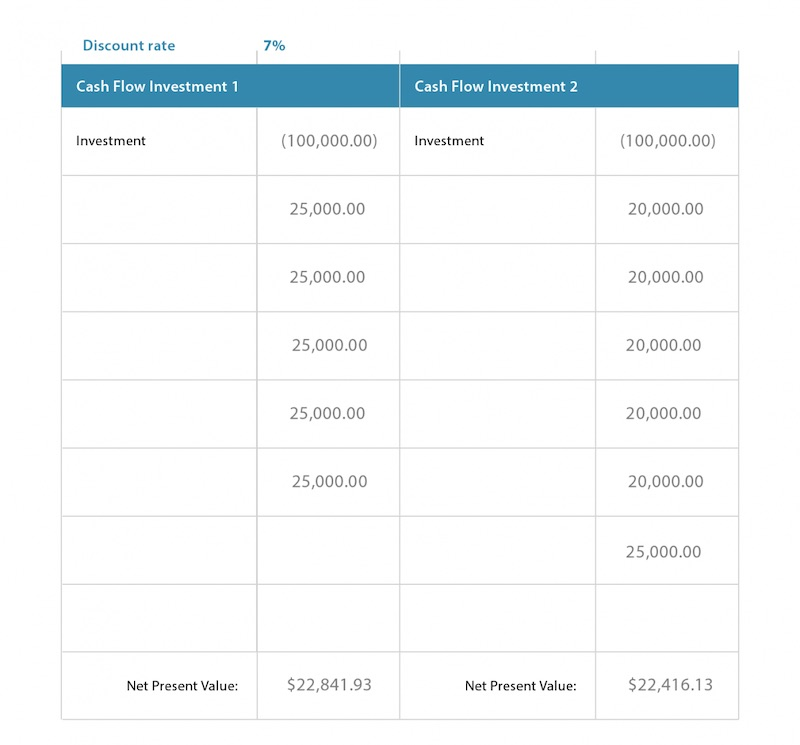# Net Present Value (NPV)

Updated November 4, 2020

## What Is Net Present Value?

Net present value (NPV) reflects a company’s estimate of the possible profit (or loss) from an investment in a project. Companies must weigh the benefits of adding projects versus the benefits of holding onto capital.

Investors often use NPV to calculate the pros and cons of investments. For example, you may wish to invest \$100,000 in a bond. One investment under consideration offers five equal payments; the other investment you’re examining offers five smaller payments (but a sixth final and larger payment). Calculating the NPV will help you determine the better investment.

## NPV Relies Upon Time Value of Money

Because the value of money changes over time, net present value must account for the time value of money – along with both its present value and future value. The benefits and losses from each time period that’s under consideration must be counted in the NPV. The analysis is conducted over a specific time period: quarterly, annually, etc. The typical  interval is one year.

### Net Present Value vs. Internal Rate of Return

The key difference between net present value vs internal rate of return is time. While both look at the profitability of an investment, the internal rate of return is calculated so that the net present value is always zero. This enables investors to compare two or more unique investments over various time periods and evaluate their profit potential. NPV takes into account the difference over time.

## How to Use Net Present Value

Net present value may be used to compare investments over a period of time or for capital budgeting projects. Because net present value takes into account the time value of money, it helps investors decide whether keeping their money or investing it will yield a better return at a future date. The net present value rule states that if a return using NPV is above 0, the project may be worth an investment.

## How to Calculate Net Present Value

To determine the net present value, each cash inflow and outflow must be discounted back to its present value. The discount value reflects the cost of capital and its risk. All of the final values are then summed up.

### Net Present Value Formula

We’re going to let you in on a big secret: No one calculates NPV by hand anymore. There’s actually an NPV function in Excel that calculates it for you.

But if you’re a student and your finance teacher insists you do at least a few calculations by hand (or you simply don’t have access to a spreadsheet program), here’s the NPV formula:Companies typically use their weighted average cost of capital (after tax) for i, the discount rate. You can also use a higher amount to account for a riskier project.

## Calculating NPV in Excel or Other Spreadsheet Programs

If calculating NPV by hand isn’t going to cut it, a spreadsheet program like Excel is preferred.

To calculate NPV using Excel:

2. Type in the discount rate.

3. Make two columns to compare your investments.

4. Type in the expected return, keeping the information sequential.

5. When you have the chart filled out, use the following formula to calculate the NPV of each investment.

6. In an empty cell, type =NPV (cell with discount rate/time period, sum of returns)

## An Example of NPV

Money that a company has in its coffers can also be used for multiple tasks like paying off loans, investing in new machinery or equipment, or hiring more workers. Calculating NPV also provides managers with a useful tool to compare the financial benefits of various projects.

The same goes for an investor considering two investment options. If you receive a windfall of \$100,000, should you put that money into Investment A or B? In this example, both Investment 1 and Investment 2 provide returns over a 12-month period. The discount rate is the same for both investments. The difference is in the number and amount of payouts over the 12-month period.

The following example shows you how to use NPV to gather all the facts before investing:Investment 1 is clearly the winner, even though six payments over a 12-month period (the numbers we used for the calculation) sounds better than five payments during the same period.

## Net Present Value Offers a Useful Gauge for Potential Investments

Net present value calculations provide a useful metric to judge two comparative investments; value over a period of time. When you’re trying to make the most from your money, knowing how much each will return – and using that as a gauge to make a final decision – minimizes  guesswork.

All of our content is verified for accuracy by Mark Herman, CFP and our team of certified financial experts. We pride ourselves on quality, research, and transparency, and we value your feedback. Below you'll find answers to some of the most common reader questions about Net Present Value (NPV).
##### Be the first to ask a question

Mark Herman, CFP - 145Mark Herman has been helping friends with financial questions since serving as an Army helicopter pilot. Since then, he’s gained valuable experience in the corporate world before moving on to become a Certified Financial Planner™.